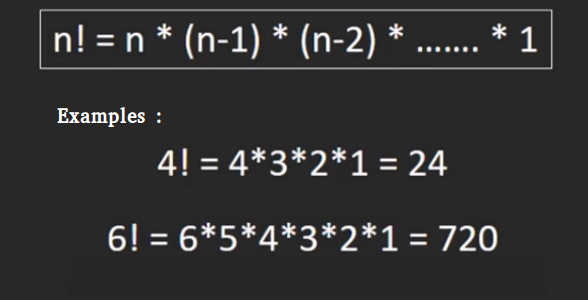# Python Program for factorial of a number

Factorial of a non-negative integer, is multiplication of all integers smaller than or equal to n. For example factorial of 6 is 6*5*4*3*2*1 which is 720.Recursive :

 `# Python 3 program to find  ` `# factorial of given number ` `def` `factorial(n): ` `     `  `    ``# single line to find factorial ` `    ``return` `1` `if` `(n``=``=``1` `or` `n``=``=``0``) ``else` `n ``*` `factorial(n ``-` `1``);  ` ` `  `# Driver Code ` `num ``=` `5``; ` `print``(``"Factorial of"``,num,``"is"``, ` `factorial(num)) ` ` `  `# This code is contributed by Smitha Dinesh Semwal `

Iterative:

 `# Python 3 program to find   ` `# factorial of given number ` `def` `factorial(n): ` `     `  `    ``# single line to find factorial ` `    ``return` `1` `if` `(n``=``=``1` `or` `n``=``=``0``) ``else` `n ``*` `factorial(n ``-` `1``);  ` ` `  `# Driver Code ` `num ``=` `5``; ` `print``(``"Factorial of"``,num,``"is"``, ` `factorial(num)) ` ` `  `# This code is contributed by Smitha Dinesh Semwal `

One line Solution (Using Ternary operator):

 `# Python 3 program to find ` `# factorial of given number ` ` `  `def` `factorial(n): ` ` `  `    ``# single line to find factorial ` `    ``return` `1` `if` `(n``=``=``1` `or` `n``=``=``0``) ``else` `n ``*` `factorial(n ``-` `1``)  ` ` `  ` `  `# Driver Code ` `num ``=` `5` `print` `(``"Factorial of"``,num,``"is"``, ` `      ``factorial(num)) ` ` `  `# This code is contributed ` `# by Smitha Dinesh Semwal. `

Please refer complete article on Program for factorial of a number for more details!

My Personal Notes arrow_drop_up
Article Tags :
Practice Tags :

7

Please write to us at contribute@geeksforgeeks.org to report any issue with the above content.